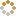﻿ N-B-U-T Online Judge ::  Rightmost Digit
• ###  Rightmost Digit

• 时间限制: 1000 ms　内存限制: 65535 K
• 问题描述
• Given a positive integer N, you should output the most right digit of N^N.
• 输入
• The input contains several test cases. The first line of the input is a single integer T which is the number of test cases. T test cases follow.
Each test case contains a single positive integer N(1<=N<=1,000,000,000).
• 输出
• For each test case, you should output the rightmost digit of N^N.

• 样例输入
• ```2
3
4```
• 样例输出
• ```7
6
```
• 提示
• ```In the first case, 3 * 3 * 3 = 27, so the rightmost digit is 7.
In the second case, 4 * 4 * 4 * 4 = 256, so the rightmost digit is 6.
```
• 来源
• `HDOJ-1061`
• 操作
﻿